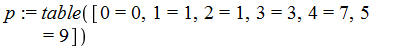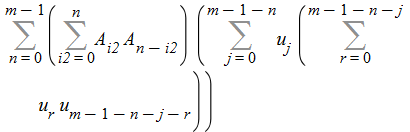Carl Love

## 26072 Reputation

11 years, 49 days
Himself
Wayland, Massachusetts, United States
My name was formerly Carl Devore.

## Attached file missing...

Your attached file is missing. But attemping to answer anyway, I get the different series:

`series(exp(1/z), z=1);                          3               2   13               3exp(1) - exp(1) (z - 1) + - exp(1) (z - 1)  - -- exp(1) (z - 1)                           2                   6                      73               4   167               5    /       6\   + -- exp(1) (z - 1)  - --- exp(1) (z - 1)  + O\(z - 1) /     24                   40                               series(exp(z), z=1);                          1               2   1               3exp(1) + exp(1) (z - 1) + - exp(1) (z - 1)  + - exp(1) (z - 1)                           2                   6                     1                4    1                5    /       6\   + -- exp(1) (z - 1)  + --- exp(1) (z - 1)  + O\(z - 1) /     24                   120                              `

## Extracting data from plots...

The data matrix for most simple plots p (including the ones here) is op([1,1], p). We can take both the x and y coordinates from the first plot and take just the y coordinate column ([..,2]) from the other two. We paste the columns together with the Matrix constructor `<|>` (which is not often used in this prefix form). Putting that all together, we get the 50 x 4 Matrix via

M:= `<|>`(op([1,1], p1), map(p-> op([1,1], p)[..,2]^%T, [p2,p3])[]);

Then you can right click on it and select Export, or use ExportMatrix(...).

Note that if you use 50 points on the interval 0..1, then the spacing is 1/49, so you're not going to get nice round x values like you show in your example.

## table, =~, sort, rand...

Did you mean p(max(m)) = max(n) rather than what you wrote, p(max(m)) = p(max(n))? If so, this will work:

m:= [\$0..5]:  n:= [\$0..9]:
M:= nops(m):  N:= nops(n):
R:= rand(N):
p:= table(m =~ [0, sort(['R()' \$ M-2])[], n[-1]]);## Sum...

Like this

Sum(Sum(A[i2]*A[n-i2], i2= 0..n)*
Sum(u[j]*Sum(u[r]*u[m-1-n-j-r], r= 0..m-1-n-j), j= 0..m-1-n),
n= 0..m-1);## Simply `/`...

Ordinary division by `/` is overloaded to work correctly with mod:

s= 2503/2275 mod 1563;
s = 976

For your other question, put the equation just as you have it into isolve:

isolve(s = (940+1563*k)/2275);
{k = 1420 + 2275 _Z1, s = 976 + 1563 _Z1}

## C-style indexing won't work right...

Maple's Matrices and multi-dimensional Arrays should be indexed SOM[i,j]. Your second attempt didn't work because you had SOM[i][j]. This latter style of indexing will work sometimes, such as when accessing the value, but it won't work for an Array or Matrix element on the left side of the assignment operator (:=). Unfortunately, it doesn't produce an error message either.

Your first attempt didn't work because your initializer wasn't a procedure. Markiyan's answer shows how to correct that.

## Use a normal plot with a procedure...

You can use the regular plot command to plot any procedure that takes a real numeric argument and returns a real numeric value. The procedure could encapsulate your fsolve call. Like this:

f:= proc(x)
local y;
fsolve(sin(y)=x, y= -Pi/2..Pi/2)
end proc:

plot(f, -0.9..0.9);

Here are some mistakes that I found:

1. You define a g (you called it "the function to iterate"), but I can't see that you ever use it.
2. You assign a plot to IP, but later on you use IP as if it were a list of points.
3. You define a list of points Initial, but I can't see that you ever use it.
4. You define CylPts as a function; it should be that function mapped over the list of points SortedPts.
5. The parameter of CylPts should be P, not IP.
6. ErrR and ErrZ should have n points, not n+1.
7. In the last line, you use a variable InitialPlot whose value was never assigned.

The big error is 2. I recommend that while you are writing code, you should not end lines with a colon. If you had been watching the output of your commands, you would've spotted error 2 much sooner.

## Concentration is just amount / volume...

Good work so far.

(ii) The concentration is just the amount of salt divided by the volume of the tank. The volume is constant at 100 liters. The amount is your answer to (i).

(iii) "Steady state" just means the limit as time t goes to infinity. The answer to that is probably obvious to you. But you can get it in Maple via limit(sol, t= infinity);

(iv) Part (iv) is to part (iii) as part (ii) is to part (i). Understand?

## combine...

In fact, Maple's square roots are the principal values, and thus Maple's square roots of positive values are always positive. The situation that you describe arises simply because Maple chooses not to do the simplification automatically. But you tell Maple to do it with combine.

`sqrt(6) - sqrt(2)*sqrt(3);                      (1/2)    (1/2)  (1/2)                     6      - 2      3     combine(%);                               0sqrt(2)*sqrt(3)*sqrt(5)*sqrt(7);                   (1/2)  (1/2)  (1/2)  (1/2)                  2      3      5      7     combine(%);                               (1/2)                            210     `

## combine...

Your expression can be entered as sin(x)^3 or (sin^3)(x), but sin^3(x) will not work. Once the expression is entered, you can use the command combine. That might seem like a strange name for this operation, but this is just a special case.

`ex:= (sin^3)(x);                                  3                            sin(x) combine(%);                      1            3                           - - sin(3 x) + - sin(x)                      4            4       `

## Solved: Minimal subcover with Dijkstra's...

I got stuck on the O(n~+ h) algorithm that I was thinking of. I couldn't figure out a way to avoid comparing every pair of intervals before applying Dijkstra's algorithm. Dijkstra's itself is O(n~+ h), n being the number of vertices (intervals in this case) and h being the number of edges (pairs of intervals that overlap in this case). Since every pair of intervals is compared (to see if they overlap), this solution in O(n2). That's okay for 1000 intervals.

This problem seems closely related to other standard problems of combinatorial optimization, such as the knapsack problem. Does anyone here know if it is equivalent to one of the standard problems? Joe Riel, I have a feeling that you may know something about this.

The solution that I was first attempting tried to find the overlapping pairs of intervals by using separate sorted lists of the lower bounds and upper bounds. I tried to use the new permutation option to sort in conjunction with this. Put succinctly, the relevant subproblem is this:

Given a set of intervals, find all pairs that overlap, using sorting rather than checking every pair.

Any ideas? Joe?

So, here's my code:

```MinimalSubcover:= module()option `Written 10 May 2013 by Carl Love.`;uses GT= GraphTheory;local    #Each interval becomes a vx in a graph. Vxs are adjacent iff their intervals overlap:    #Let I1 = (a1,b1) and I2 = (a2,b2) be intervals. There is an edge I1 -> I2 iff    # a1 < a2 < b1 < b2.    Edges,  #Table of edges    EdgeCt, #Integer index to the table    Lower,  #List of lower bounds of intervals    Upper,  #List of upper bounds of intervals    #Compute an edge's weight and add it to the table. An edge's weight is the average    #of the weights of its vxs. A vx's weight is the upper bound of its interval minus    #the lower bound. The special vertices "Start" and "End" have weight 0.    AddEdge:= proc(e::list)    local w; # edge weight        if e="Start" then  w:= Upper[e] - Lower[e]        elif e="End" then  w:= Upper[e] - Lower[e]        else  w:= Upper[e]-Lower[e] + Upper[e]-Lower[e]        end if;        EdgeCt:= EdgeCt+1;        Edges[EdgeCt]:= [e, w/2];        [][]    end proc,    ModuleApply:= proc(Cover::{list,set}, ClosedInterval::specfunc(numeric, RealRange))    local        A:= op(1, ClosedInterval),        B:= op(2, ClosedInterval),        # Remove duplicates.        # Force to a list.        # Remove any intervals that do not intersect the target interval.         Subcover:= remove(OI-> op([1,1], OI) >= B or op([2,1], OI) <= A, [{Cover[]}[]]),        N:= nops(Subcover),        k, k1, k2, Soln    ;                 Edges:= table();         EdgeCt:= 0;         Lower:= map(x-> op([1,1], x), Subcover);        Upper:= map(x-> op([2,1], x), Subcover);        #Intervals with lower bounds less than the lower bound of the target interval get        #connected to special vx "Start". Those with upper bounds greater than the upper        #bound of the target interval get connected to "End".        for k to N do             if Lower[k] < A then  AddEdge(["Start", k])  fi;            if Upper[k] > B then  AddEdge([k, "End"])  fi        end do;       #Check each pair of intervals for overlap.        for k in combinat:-choose(N,2) do            (k1,k2):= k[];            if Lower[k1] < Lower[k2] and Lower[k2] < Upper[k1] and Upper[k1] < Upper[k2] then                AddEdge([k1,k2])            elif Lower[k2] < Lower[k1] and Lower[k1] < Upper[k2] and Upper[k2] < Upper[k1] then                AddEdge([k2,k1])            fi        end do;        try                    #Compute shortest path through graph.             Soln:= GT:-DijkstrasAlgorithm(GT:-Graph({entries(Edges, nolist)}), "Start", "End");             Soln, Subcover[Soln[2..-2]]        catch:             infinity, []        end try    end proc;end module: ------------------------------------------------------------------------------------------------------Sorry, I don't know how to get rid of the extra blank lines below. They do not appear in the editor when I am creating the post.

>

>

G:= RandomTools:-Generate(makeproc, float(range= 0..10^4, digits= 4)):

>

L:= ['RealRange('Open(G())' \$ 2)' \$ 2*10^3]:

>

M:= remove(`=`, L, BottomProp):

>

nops(M);>

st:= time():

>

MinimalSubcover(M, RealRange(1., 1000.));>

time()-st;>

## The initial square is a different case...

It's the same situation as with the Koch snowflake: The initial square has no left turns: "FRFRFRFR" (or, as one may say in Maple, cat("FR"\$4)). We replace every F by the recursion to get

cat(cat(Box(n), "R") \$ 4);

You are correct that the U shape is the recursive "unit": "FRFLFLFRF". Note that there's no "R" on the end of that. Now replace every F in that with recursion.## RootFinding:-NextZero...

For a non-polynomial equation, fsolve gives just one solution. One of the best ways to get all the roots of an analytic function in an interval of reals is to use RootFinding:-NextZero:

`r:= -5:for k while r < 5 do   r:= RootFinding:-NextZero(x-> sin(Pi*x^2/2), r);   Sols[k]:= rend do:k:= k-2; # Number of solutions                               25seq(Sols[i], i= 1..k);-4.89897948556636, -4.69041575982343, -4.47213595499958,   -4.24264068711928, -4.00000000000000, -3.74165738677394,   -3.46410161513776, -3.16227766016838, -2.82842712474619,   -2.44948974278318, -2.00000000000000, -1.41421356237310, 0.,   1.41421356237310, 2.00000000000000, 2.44948974278318,   2.82842712474619, 3.16227766016838, 3.46410161513776,   3.74165738677394, 4.00000000000000, 4.24264068711928,   4.47213595499958, 4.69041575982343, 4.89897948556636`

## Try something more complex (not just lar...

I don't know the exact reason that your example doesn't show any benefit. I do duplicate your results on my machine. I also randomized the list order and still got no better results from Threads. It may be that the task (computing the 10th power of an integer less than 10^6) is too trivial. Here's an example where there is a clear benefit to using Threads:

`restart;L:= RandomTools:-Generate(list(posint, 2^16)):CodeTools:-Usage(map(nextprime, L)):memory used=2.25GiB, alloc change=2.00MiB, cpu time=21.17s, real time=21.20srestart;L:= RandomTools:-Generate(list(posint, 2^16)):CodeTools:-Usage(Threads:-Map(nextprime, L)):memory used=2.25GiB, alloc change=71.34MiB, cpu time=52.78s, real time=8.38sDone on an i7 3630QM 4x2.4 GHz`
﻿